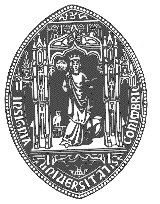7th European Intensive Course on Complex Analysis

“Complex Analysis and its Generalizations (with applications to partial differential equations)”

Departamento de Matemática, Universidade de Coimbra, Portugal

19-30 March 2001

With support from CMUC (Centro de Matemática da Universidade de Coimbra), UI&D "Matemática e Aplicações" da Universidade de Aveiro, and the Socrates programme

 Goal of the Course Schedule of the course Abstracts History Financial Support Organizers Coming Events Registration Form

#### Goal of the Course

This intensive course will have a total of 40 hours of lectures and is at postgraduate level. Lecturers will have time available to discuss with the students. Successfully participating students will get a certificate. This course is organized by the Universities of Coimbra and Aveiro with the same goals as the ones organized under the programme Socrates, and is open to all young mathematicians interested in Complex Analysis and its applications.

There will be an Workshop on "Applications and Generalizations of Complex Analysis" on the 23th and 24th of March.#### Schedule of the course19 March 20 March 21 March 22 March 23 March Opening session 9h-9h30m * * * * 9h30m-12h30m 9h-12h30m 9h-12h30m * * Laine 15h -17h 15h -17h * 10h -12h ------------- 15h - 17h 10h -12h
 26 March 27 March 28 March 29 March 30 March 10h-12h 10h-12h Student Presentation 10h-12h 10h-12h 15h -17h 15h -17h * 15h -17h 15h -17h

#### Abstracts

OPERATOR THEORY AND MOMENT PROBLEMS
- Francisco Marcellán (Univ. Carlos III, Madrid, Spain)

Abstract: The aim of this course is to introduce the state-of-the art of moment problems from the perspective of the theory of finite difference operators in the scalar and in the matrix case, respectively. One the advantages of this approach is that the Nevanlinna functions appear as elements of a transfer matrix and the Padé approximants are the resolvents of a finite matrix approximation to a infinite Jacobi matrix.

Contents:

1. Spectral theorem for bounded and unbounded self-adjoint operators.
2. Orthogonal polynomials and Jacobi operators. Doubly infinite Jacobi operators.
3. The Hamburger and the Stieltjes moment problems as self-adjointness problems.
4. Transfer matrices and the indeterminate moment problem.
5. Padé Approximants, continued fractions and finite matrix approximations. Solutions of finite order.

Basic references that will be distributed to the participants in the course.
.- A. I. Aptekarev, E. M. Nikishin, The scattering problem for a discrete Sturm-Liouville operator, Mat. USSR Sbornik 49 (1984) 325-355.
.- B. Beckermann, Complex Jacobi matrices. Journal of Computational and Applied Mathematics. 2001. To appear.
.- B. Simon, The classical Moment Problem as a Self Adjoint Finite Difference Operator, Advances in Mathematics 137 (1998) 82-203INTRODUCTION TO COMPLEX DIFFERENTIAL EQUATIONS
- Ilpo Laine (Univ. Joensuu, Finland)

Abstract: These lectures offer a short introduction to differential equations in the complex domain through the following subtitles: Local existence theorems, linear differential equations (theorem of Wittich, basic value distribution of solutions), oscillation properties of solutions of f" + A(z)f = 0, first order algebraic differential equations (theorems of Gol'dberg and Malmquist), Riccati differential equation, Painlevé differential equations
(meromorphic nature of solutions and their value distribution), differential equations in the unit disk.CONFORMAL INVARIANCE IN CLIFFORD ANALYSIS
- Vladimir Soucek (Charles University, Czech Republic)

Abstract: The series of lecture will contain a discussion of simplest conformally invariant first order differential equations and basic properties of their solutions. The principle example will be the Dirac operator on domains in Euclidean space. The main points of the series of lectures can be summarized as follows:

1. Clifford algebras in even and odd dimensions, the spinor group and classification of its representations by highest weights.
2. The group G of conformal transformations, the sphere as its homogeneous space, stereographic projection, the group CO(n) and its representations.
3. Homogeneous spaces (Klein's Erlangen program), homogeneous bundles and invariant operators on the spaces of their sections. The Fegan construction of invariant first order differential operators.
4. The Dirac operator and its conformal invariance.
5. Spherical monogenics and their interpretation as Spin(n) irreducible modules.
6. The twisted Dirac operator, the Rarita-Schwinger operator and its symmetric analogues, their conformal invariance.
7. Monogenic functions of two Clifford variables and their connections with the generalized Rarita-Schwinger operators.AN INTRODUCTION TO SEVERAL COMPLEX VARIABLES
- Chris Boyd (Univ. College, Dublin, Ireland)

Abstract: We begin by showing how certain classical results from one complex variable (e.g. The Open Mapping Theorem, The Identity Principle and Montel's Theorem) generalise to several complex variables. The
Riemann Mapping Theorem is one of the fundamental results of single variable complex analysis. We will show that for n >= 2 this result is no longer true. To obtain this result we examine groups of biholomorphic mappings on domains in Cn. In the process of our investigations we obtain results such as Cartan's Uniqueness Theorem and Poincar\'e's Theorem for the Polydisc.• • Student presentations

29 March: 10-12h#### History

This intensive course follows the six held in Coimbra and Aveiro from 1995 to 1999 and there are plans for intensive courses in the following years. The lecture notes of some of the courses have been published in Coimbra and others are in print.#### Financial Support

Living expenses can be partially covered for some students if they do not have support from their own institution and if there is enough money available.Coming events